# Tips To Prepare NSEP Physics Chapter On Alternating Current

###### BySafeer PP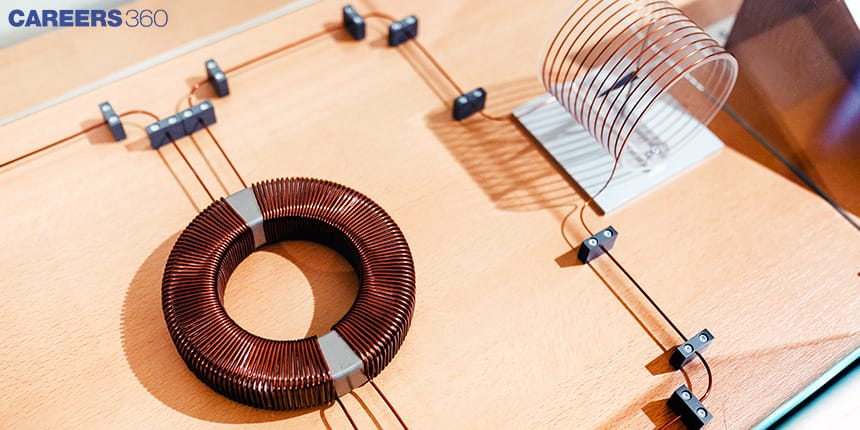###### Synopsis

Indian Association of Physics Teachers organises National Standard Examination In Physics. Student toppers from NSEP can attend Indian National Olympiad In Physics. To crack NSEP it is important to understand how to prepare the chapters of the NSEP syllabus. Read on to know how to prepare the chapter Alternating Current for NSEP###### Synopsis

Indian Association of Physics Teachers organises National Standard Examination In Physics. Student toppers from NSEP can attend Indian National Olympiad In Physics. To crack NSEP it is important to understand how to prepare the chapters of the NSEP syllabus. Read on to know how to prepare the chapter Alternating Current for NSEP

Alternating Current is an important portion of the NSEP syllabus as questions are frequently asked from this chapter. Let’s discuss the NSEP previous year questions for practice, tips, some important points, formulas and study materials to prepare Alternating Current for NSEP.

Also Read| Previous Years Paper Analysis For NSEP

## Tips To Prepare Alternating Current For NSEP

• Understand the basic terms line instantaneous value, peak value, average value and RMS value.

• Understand the phasor diagram for AC applied to an inductor, capacitor and RLC series circuit.

• Solve problems on RLC circuits, series resonance and LC oscillations.

## Important Points To Remember From Alternating Current

• The current in a pure inductor circuit lags voltage by 90 degrees.

• Pure inductor in DC circuit act as a short circuit.

• A fully charged capacitor appears as an open circuit in DC.

• The current in a pure capacitor circuit lead voltage by 90 degrees.

• Power factor at resonance =1 and the current is maximum as impedance is minimum. Z=R.

## Alternating Current Important Formulas

The following formulas may help in the preparation of NSEP.

### Average Value

Average value = area under the curve/length of the base of the curve

Or the average value of voltage is given by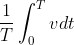Where v is the instantaneous value of alternating voltage

### RMS Value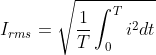i is the instantaneous value of alternating current

## LCR Series Circuit

### Resultant Voltage Of LCR Series Circuit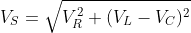### Impedance Z Of LCR Series Circuit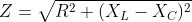As XL=⍵L and XC=1/(⍵C)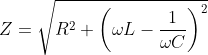### Power In Series LCR Circuit

Instantaneous Power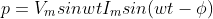Average Power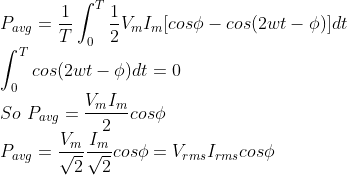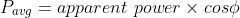### Power Factor

The power factor cosɸ can be calculated as-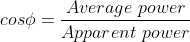### Resonant Frequency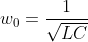Q=⍵0L/R

Q=1/(⍵0RC)

### BandWidth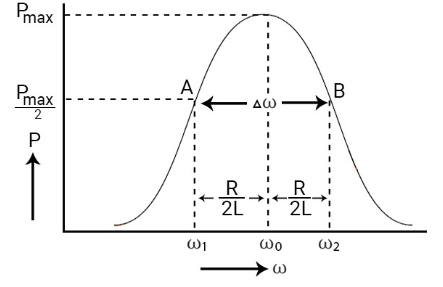Bandwidth =R/L

## Alternating Current NSEP Previous Year Questions

Q-1- A 10 ohm resistor is connected to a supply voltage alternating between + 4V and – 2V as shown in the following graph. The average power dissipated in the resistor per cycle is (NSEP 2017)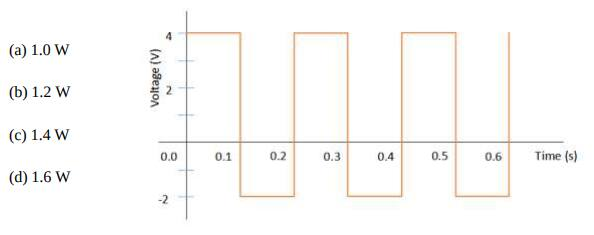Q-2- In the following circuit, the current is in phase with the applied voltage. Therefore, the current in the circuit and the frequency of the source voltage respectively, are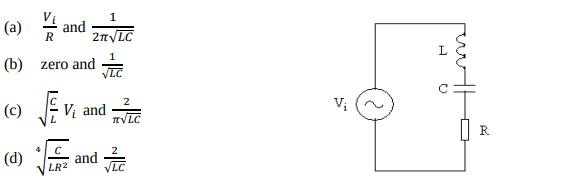Q-3- ) In a series R-C circuit the supply voltage (Vs) is kept constant at 2V and the frequency f of the sinusoidal voltage is varied from 500 Hz to 2000 Hz. The voltage across the resistance R = 1000 ohm is measured each time as VR. For the determination of the C a student wants to draw a linear graph and try to get C from the slope. Then she may draw a graph of(NSEP 2017- Morethan one correct option)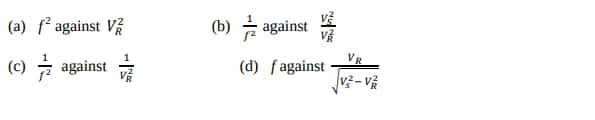Q-4- In a series LCR circuit fed with an alternating emf E = E0 sin ωt(NSEP 2018)

(a) the voltage across L is in phase with the applied emf E.

(b) the voltage across C is in phase with the applied emf E.

(c) the voltage across R is in phase with the applied emf E.

(d) the voltages across L, C and R are all in phase with the applied emf E.

Q-5- After charging a capacitor C to a potential V, it is connected across an ideal inductor L. The capacitor starts discharging simple harmonically at time t = 0. The charge on the capacitor at a later time instant is q and the periodic time of simple harmonic oscillations is T. Therefore, (NSEP 2018 - More than one correct answer)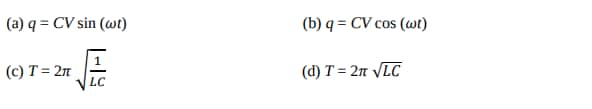Q-6- An inductance L, a resistance R and a battery B are connected in series with a switch S. The voltages across L and R are VL and VR respectively. Just after closing the switch S (NSEP 2018 - More than one correct answer)

(a) VL will be greater than VR.

(b) VL will be less than VR.

(c) VL will be the same as VR.

(d) VL will decrease while VR will increase as time progresses.

Q-7- The switch S in the circuit shown is closed for a long time and then opened at time t = 0. The current in the 100 kΩ resistance at t = 3s is (NSEP 2018)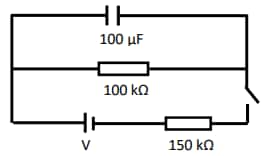(a) zero.

(b) 48 µA.

(c) 35.5 µA.

(d) 16 µA.

Q-8- In an experiment with a potentiometer, the balancing length is 250 cm for a cell. When the cell is shunted by a resistance of 7.5 W, the balancing point is shifted by 25 cm. If the cell is shunted by a resistance of 20W, the balancing length will be nearly (NSEP 2020)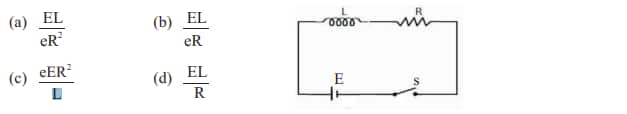(a) 240 cm (b) 236 cm (c) 232 cm (d) 230 cm

## NSEP Study Material For Alternating Current

• NCERT Class 12 Physics Chapter Alternating Current

• NCERT Exemplar problems on Alternating Current

• NSEP previous year question papers

• Technology
• Computer engineering
• Electromagnetism
• Electricity
• Electrical engineering
• Physical quantities
• Metrology
• Quantity
• Electronics
• Electronic engineering

## Careers360 helping shape your Career for a better tomorrow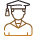#### 250M+

Students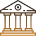#### 30,000+

Colleges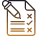#### 500+

Exams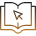E-Books